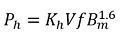# Difference Between Eddy Current & Hysteresis Loss

The most significant difference between the Eddy current and Hysteresis loss is that the eddy current loss occurs because of the relative motion between the conductor and the magnetic field. Whereas the hysteresis loss occurs because of the reversal of the magnetism. The other differences between the hysteresis and the eddy current loss are explained below in the comparison chart.

## Comparison Chart

Basis for Comparison Eddy current LossHysteresis Loss
DefinitionThe loss occur because of the relative motion between the core and the magnetic flux.The losses which occur because of the reversal of the magnetism is known as the hysteresis loss.
FormulaOccurInteraction of the magnetic flux and conductor. Because of reversal of flux.
Minimising Method

By making the core of thin lamination. By using Silicon Steel Material

### Definition of Hysteresis Loss

The hysteresis loss occurs in the core of the transformer. The core of the transformer is made of the CRGO steel which is a type of ferromagnetic material. The ferromagnetic material is made by the small atomic magnetic moments. When no field is applied to the material, in that case, the molecules are randomly arranged in the substance.

When the MMF force applied to the material then the atomic magnetic moment arranged themselves parallel to each other in the axis of the magnetomotive force.When force removes then the atomic magnetic moment again arranges themselves randomly but few of them still align parallel to each other. Because of these atomic magnetic moments the substance becomes slightly magnetizes. This type of magnetism is known as the spontaneous magnetism.

For removing the spontaneous magnetism the reversed MMF applied to the material. The losses which occur because of the reversal of magnetism is known as the hysteresis losses.

### Definition of Eddy Current Loss

The eddy current induces in the core of the transformer when the alternating flux links with them. The flux induces the EMF in the core because of which the circulating current develops. The circulating current cause losses in terms of heat which increases the temperature of the coil.The eddy current losses are independent of load and hence known as the constant losses.

## Key Differences between the Hysteresis and Eddy Current Loss

1. The loss which occurs because of the eddy current is known as the eddy current loss. The eddy current induces because of the interaction of the variable magnetic field and conductor. The loss which occurs because of the reversal of the magnetising force is known as the hysteresis loss.
2. The eddy current loss occurs because of the interaction of magnetic field and conductor. The hysteresis loss occurs because of the reversal of the magnetism.
3. The eddy current loss is minimised by using the thin core of lamination. The silicon steel material is used for minimising the hysteresis loss.

### Similarities

The eddy current and hysteresis loss both occurs in the core of the transformer. Because of these losses the power dissipated in the form of heat. And this heat increases the temperature of the machine.